# Napier's Bones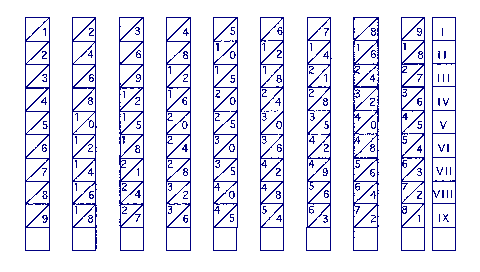Napier's bones were described in his book Rabdologie, which was published in 1617, shortly after his death. Although the bones often seem to be confused with his logarithms, there is in fact no connection and the bones represent an application of the old Arabic lattice method of multiplication. The bones consist of a series of numbered rods, each inscribed with a multiplication table; e.g. the second rod contains the two times table, with the units inscribed below the diagonal and the tens above it. There is also a guide rod, which is marked with Roman numerals as shown. The rods were usually made of ivory or bone, hence the name.

The bones took a lot of the pain out of the process of multiplication. For example, suppose we want to perform the following multiplication:-

```          628 x 427
```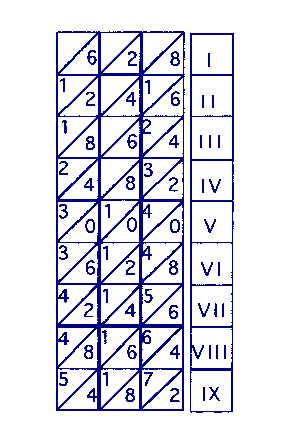We would take out the 6 the 2 and the 8 rods and lay them next to the marker rod as shown. Now remember that to multiply by 427 we have to multiply by 4 hundreds, 2 tens and 7 units.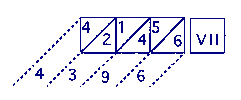Start by multiplying by the seven units. Look along the 7th row (VII) and write down the result, adding along the diagonals.

```              6 = 6
5 + 4 = 9
1 + 2 = 3
4 = 4
```

The result for the units multiplication is thus 4396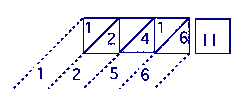Next, multiply by the two tens. Look along the 2nd row and write down the result as before.

```              6 = 6
1 + 4 = 5
2 = 2
1 = 1
```

The result for the tens multiplication is thus 12560 - (remember that the result has to be multiplied by 10)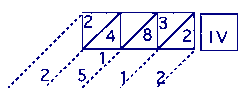Then, multiply by the four hundreds. Look along the 4th row - (this is complicated by a carry).

```              2 =     2
8 + 3 =     1  (carry 1)
4 + 1 = 5
2 =     2
```

The result for the hundreds multiplication is thus 251200 (remember the result has to be multiplied by 100)

Finally, add the intermediate results:-

```         4396
12560
251200
======
268156
======
```

The above description rather labours the point and in practice the end result can be produced quite quickly. Strictly speaking, the bones do not act as a calculating device in the modern sense of the word but merely serve to enforce the correct use of the lattice algorithm.

28-Jan-2002
Images and Text Copyright © 2002 A. Audsley, All Rights Reserved